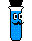# Percent by Mass

## Strategy:

1. Assume one mole of phosporus trichloride. Find the moles of phosphorus and moles of chlorine.

2. Convert mole of each element to mass of each element.

3. Calculate the percent by mass of each element.

#### Show completed problemGen Chem Topic ReviewGeneral Chemistry Help Homepage